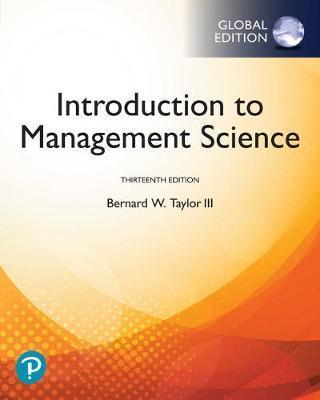# INTRODUCTION TO MANAGEMENT SCIENCE 13E GE - TAYLOR III 9781292263045

RM 117.00

TITLE : INTRODUCTION TO MANAGEMENT SCIENCE  - TAYLOR III

ISBN13 : 9781292263045

PUBLISHER : PEARSON EDUCTION (2019)

EDITION : 13E PAPERBACK

PAGES : 864  PAGES

Introduction to Management Science gives students a strong foundation in how to make decisions and solve complex problems using both quantitative methods and software tools. In addition to extensive examples, problem sets, and cases, the 13th Edition incorporates Excel 2016 and other software resources, developing students' ability to leverage the technology they will use throughout their careers. By practicing these modelling techniques, students gain a useful framework for problem-solving that they can then apply in the workplace.

1. Management Science
2. Linear Programming: Model Formulation and Graphical Solution
3. Linear Programming: Computer Solution and Sensitivity Analysis
4. Linear Programming: Modeling Examples
5. Integer Programming
6. Transportation, Transshipment, and Assignment Problems
7. Network Flow Models
8. Project Management
9. Multicriteria Decision Making
10. Nonlinear Programming
11. Probability and Statistics
12. Decision Analysis
13. Queuing Analysis
14. Simulation
15. Forecasting
16. Inventory Management

Appendix A: Normal and Chi-Square Tables
Appendix B: Setting Up and Editing a Spreadsheet
Appendix C: The Poisson and Exponential Distributions

Solutions to Selected Odd-Numbered Problems

The following items can be found on the companion website that accompanies this text:
Module A: The Simplex Solution Method
Module B: Transportation and Assignment Solution Methods
Module C: Integer Programming: The Branch and Bound Method
Module D: Nonlinear Programming Solution Techniques
Module E: Game Theory
Module F: Markov Analysis